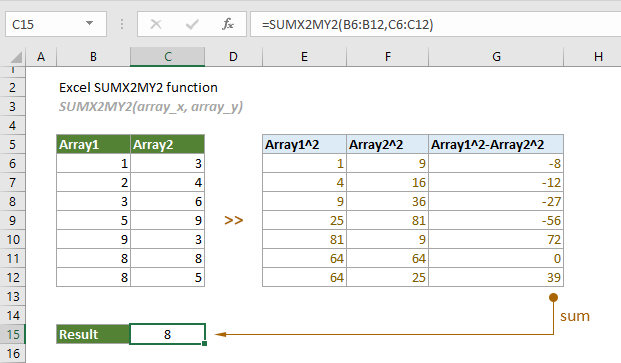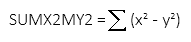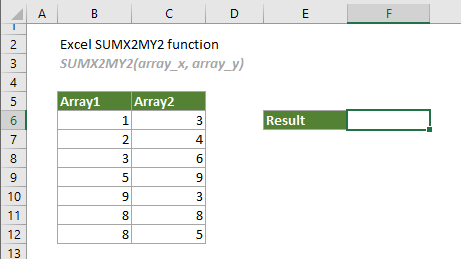## Excel SUMX2MY2 function

The SUMX2MY2 function returns the sum of the difference of squares of corresponding values in two given arrays.#### Syntax

SUMX2MY2(array_x, array_y)

#### Arguments

• Array_x (required): The first array or range of values used in the calculation;
• Array_y (required): The second array or range of values used in the calculation.

#### Remarks

1.Either of the following elements can be used in the supplied arguments:
-- Numbers;
-- Names, arrays or references that contain numbers.
2. If there are text, logical values or empty cells in the supplied array, they will be ignored;
3. Zero values will be included in the calculation；
4. The array_x and array_y must contain exactly the same number of values, otherwise, the #N/A! error value will be returned;
5. The equation of the SUMX2MY2 function is as follows:#### Return value

It returns a numeric value.

#### Example

As shown in the screenshot below, to get the sum of the differences of squares of array1 and array2, you can apply the SUMX2MY2 function as follows to handle it.1. Select a blank cell, copy or enter the formula below and press the Enter key to get the result.

=SUMX2MY2(B6:B12,C6:C12)Notes:

1. The above result can be broken down as:

=(1^2-3^2)+(2^2-4^2)+(3^2-6^2)+(5^2-9^2)+(9^2-3^2)+(8^2-8^2)+(8^2-5^2)

2. You can directly use the cell values in the formula as follows.

=SUMX2MY2({1,2,3,5,9,8,8},{3,4,6,9,3,8,5})

#### Related Functions

Excel SUMX2PY2 function
The SUMX2PY2 function returns the sum of squares of corresponding values in two given arrays.

Excel SUMXMY2 function
The SUMXMY2 function returns the sum of squares of the differences of corresponding values in two given arrays.

### The Best Office Productivity Tools

#### Kutools for Excel - Helps You To Stand Out From Crowd

Would you like to complete your daily work quickly and perfectly? Kutools for Excel brings 300 powerful advanced features (Combine workbooks, sum by color, split cell contents, convert date, and so on...) and save 80% time for you.

• Designed for 1500 work scenarios, helps you solve 80% Excel problems.
• Reduce thousands of keyboard and mouse clicks every day, relieve your tired eyes and hands.
• Become an Excel expert in 3 minutes. No longer need to remember any painful formulas and VBA codes.
• 30-day unlimited free trial. 60-day money back guarantee. Free upgrade and support for 2 years.#### Office Tab - Enable Tabbed Reading and Editing in Microsoft Office (include Excel)

• One second to switch between dozens of open documents!
• Reduce hundreds of mouse clicks for you every day, say goodbye to mouse hand.
• Increases your productivity by 50% when viewing and editing multiple documents.
• Brings Efficient Tabs to Office (include Excel), Just Like Chrome, Firefox, And New Internet Explorer.